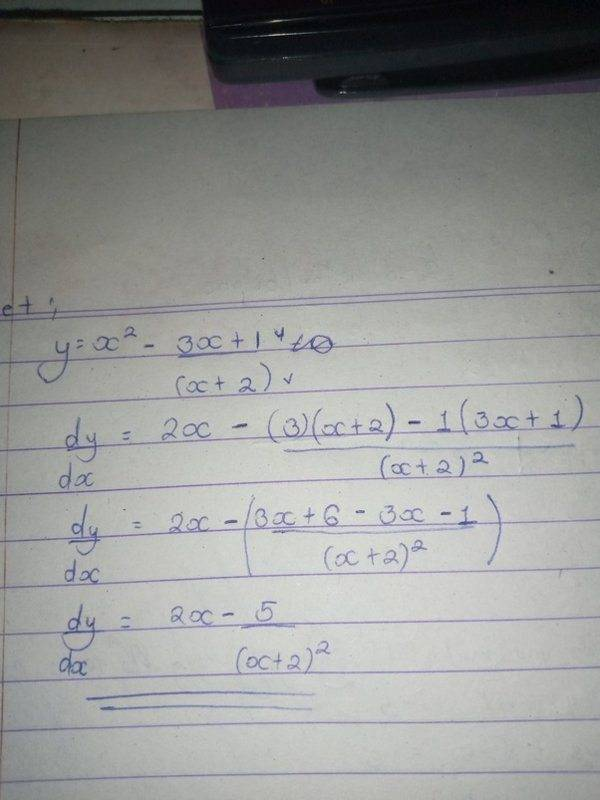# Differentiate x^2-(3x+1)/(x+2)

Homework Statement:
To be differentiated: x^2-3x+1/x+2
Relevant Equations:
n/aIs this correct?

Last edited by a moderator:

benorin
Homework Helper
Yes

•berkeman
berkeman
Mentor
Looks okay to me. Your handwriting of the math is a little hard for me to read, but it seems correct.

BTW, there is a good LaTeX tutorial at the top of the page under INFO/Help (and in the lower left of the edit window below). We encourage all users to use LaTeX when posting math at the PF, since it is so much easier for folks to read and respond to. For example, I think this is your problem and your final solution (you can use the Reply button to see how LaTeX was used to generate the equations):

$$y(x) = x^2 - \frac{3x+1}{x+2}$$
$$\frac{dy(x)}{dx} = 2x - \frac{5}{(x+2)^2}$$

•benorin
Mark44
Mentor
To be differentiated: x^2-3x+1/x+2
Don't write it this way. What you wrote would be interpreted to mean this:
##x^2 - 3x + \frac 1 x + 2##, which I'm sure isn't what you meant.
If you write a fraction using inline text, but sure to add parentheses whenever the numerator and/or denominator have multiple terms. You example should be written like this: x^2 - (3x + 1)/(x + 2).

Better yet, like this using TeX: ##x^2 - \frac{3x + 1}{x + 2}##

•FactChecker
FactChecker